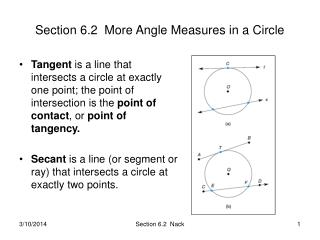DownloadDownload PresentationSection 6.2 More Angle Measures in a Circle

# Section 6.2 More Angle Measures in a Circle

Download Presentation## Section 6.2 More Angle Measures in a Circle

- - - - - - - - - - - - - - - - - - - - - - - - - - - E N D - - - - - - - - - - - - - - - - - - - - - - - - - - -
##### Presentation Transcript

1. Section 6.2 More Angle Measures in a Circle • Tangent is a line that intersects a circle at exactly one point; the point of intersection is the point of contact, or point of tangency. • Secant is a line (or segment or ray) that intersects a circle at exactly two points. Section 6.2 Nack

2. Polygons Inscribed in a Circle • A polygon is inscribed in a circle if its vertices are points on the circle and its sides are chords of the circle. • Equivalent statement: A circle is circumscribed about the polygon • Cyclic polygon: the polygon inscribed in the circle. • Theorem 6.2.1: If a quadrilateral is inscribed in a circle, the opposite angles are supplementary. Section 6.2 Nack

3. Polygons Circumscribed about a circle • A polygon is circumscribed about a circle if all sides of the polygon are line segments tangent to the circle; the circle is said to be inscribed in the polygon. Section 6.2 Nack

4. Measure of an Angle Formed by Two Chords that Intersect Within a Circle • Theorem 6.2.2: The measure of an angle formed by two chords that intersect within a circle is one-half the sum of the measures of the arcs intercepted by the angle and its vertical angle. 1. Proof: Draw CB. Two points determine a line 2. m1 = m2 + m3 1 is an exterior  of ΔCBE. 3. m2 = ½ mDB 2 and 3 are inscribed m3 = ½ mAC  ‘s of O. 4. m1 = ½ (mDB + mAC) Substitution Section 6.2 Nack

5. Theorems with Tangents and Secants • Theorem 6.2.3: The radius or diameter drawn to a tangent at the point of tangency is perpendicular to the tangent at that point. See Fig. 6.29 (a,b,c) Ex. 2 p. 289 • Corollary 6.2.4: The measure of an angle formed by a tangent and a chord drawn to the point of tangency is one-half the measure of the intercepted arc. Example 3 p. 289 Section 6.2 Nack

6. Measures of Angles formed by Secants. • Theorem 6.2.5: The measure of an angle formed when two secants intersect at a point outside the circle is one-half the difference of the measures of the two intersecting arcs. Given: Secants AC and DC Prove: mC = ½(m AD – m BE) • Draw BD to form ΔBCD • m1 = mC + mD Exterior angles of ΔBCD mC = m1 - mD Addition Property • m1 = ½m AD Inscribed Angles mD= ½ m BE • mC= ½m AD - ½ m BE Substitution mC= ½(m AD – m BE) Distributive Law Section 6.2 Nack

7. Theorem 6.2.6: If an angle is formed by a secant and a tangent that intersect in the exterior of a circle, then the measure of the angle is one-half the difference of the measure of its intercepted arcs. Theorem 6.2.7: If an angle is formed by two intersecting tangents, then the measure of the angle is one-half the difference of the measures of the intercepted arcs. Section 6.2 Nack

8. Parallel Chords • Theorem 6.2.8: If two parallel lines intersect in a circle, the intercepted arcs between these lines are congruent. AC  BD A B C D Section 6.2 Nack

9. Location of the Vertex of the Angle Center of the Circle In the interior of the circle On the Circle In the exterior of the circle Example 6 p. 292 Rule for Measuring the Angle The measure of the intercepted arc. One-half the sum of the measures of the intercepted arcs. One-half the measure of the intercepted arc. One-half the difference of the measures of the two intercepted arcs. Summary of Methods for Measuring Angles Related to a Circle. Section 6.2 Nack